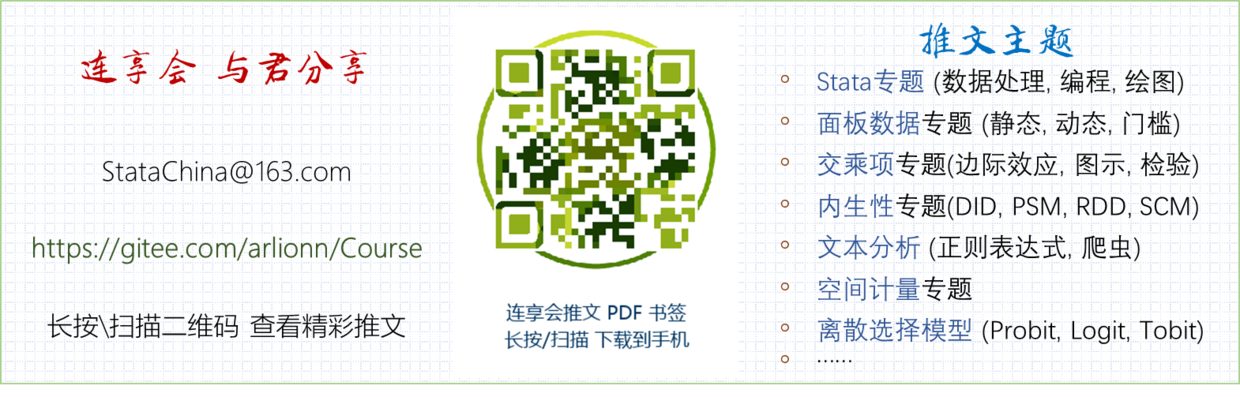## 一文看尽 Stata 绘图

Stata连享会   计量专题 || 精品课程 || 简书推文 || 公众号合集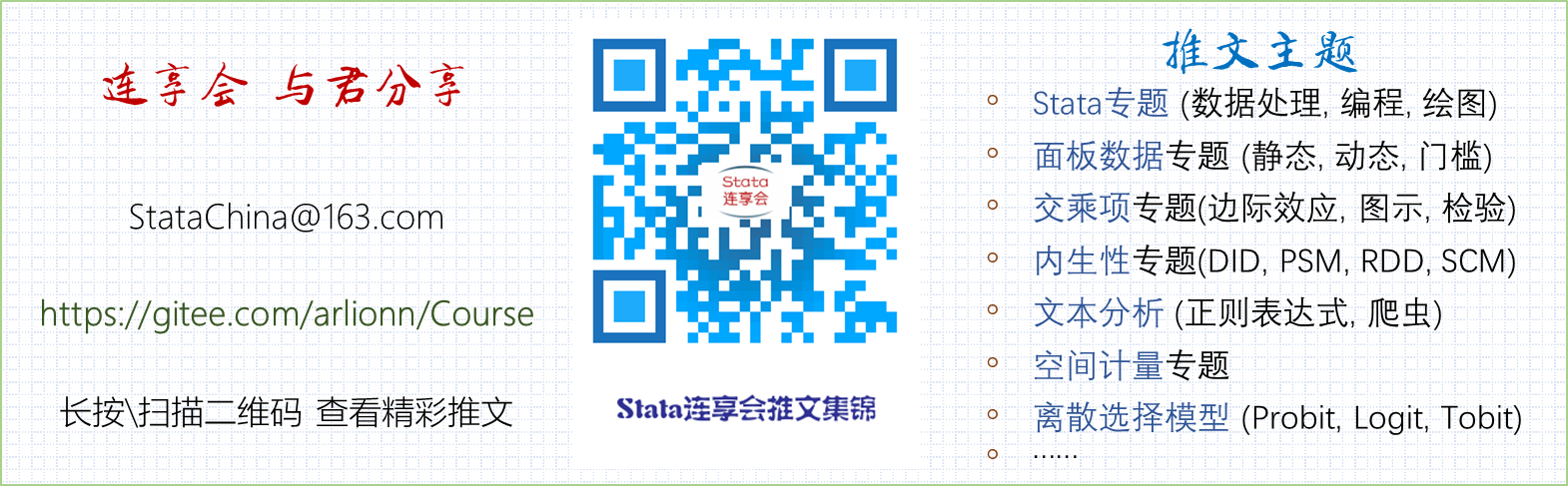### 1. 绘图简介

Stata 提供各种绘图命令，包括点 (scatter)、线 (line)、面 (area)，直方图 (histogram)、条形图 (bar)、饼图 (pie)、函数曲线 (function) 以及矩阵图 (matrix) 等。对时间序列数据有以 ts 开头的一系列特殊命令，如 tsline。对面板数据有以 xt 开头的特殊命令，如 xtline。还有一类是对双变量的回归拟合图 (lfit、qfit 、lowess 等)。具体内容可参考帮助命令 `help graph``help twoway`

#### 1.1 绘图区域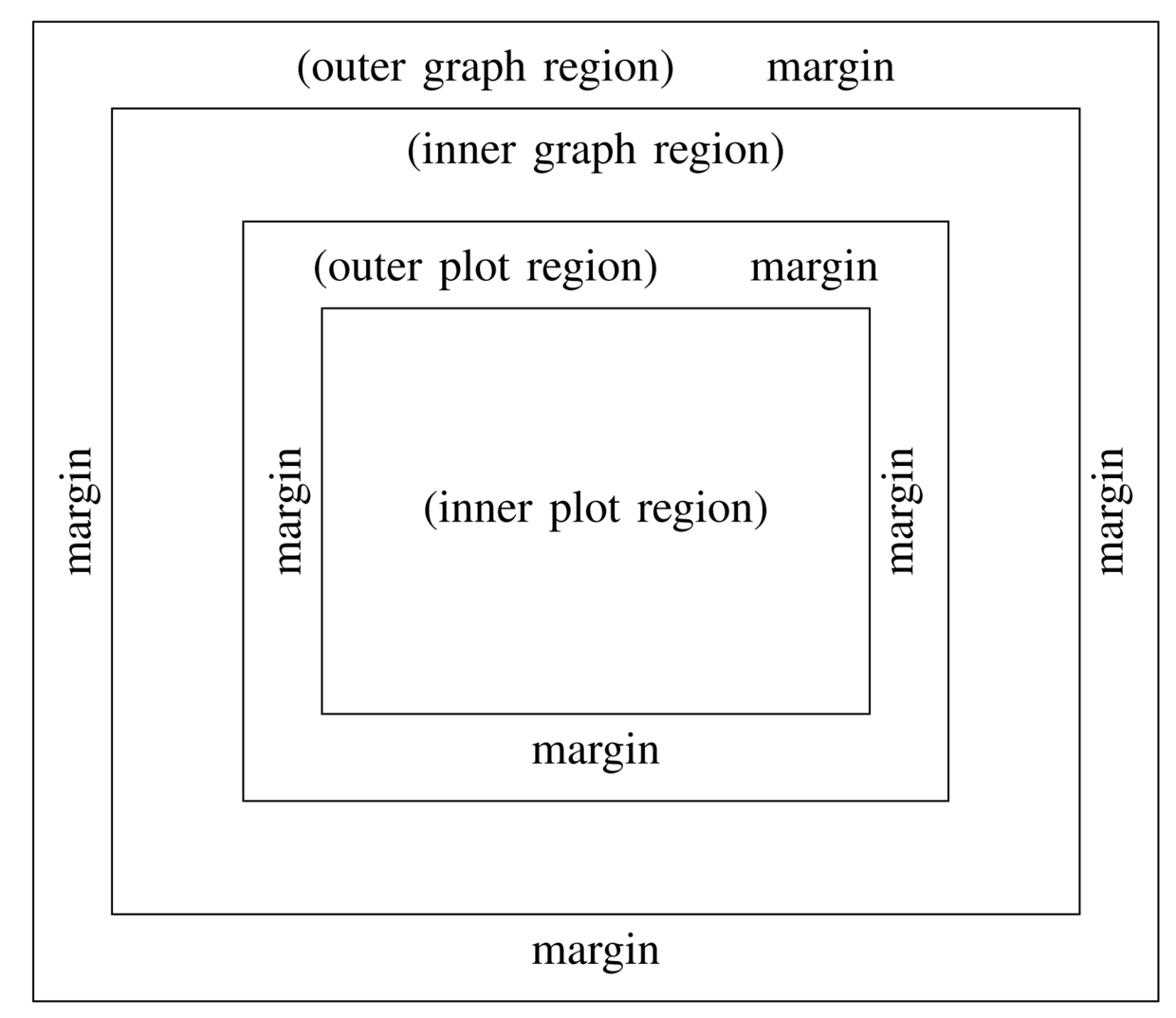#### 1.2 语法结构

``````graph-command (plot-command, plot-options) (plot-command, plot-options) (...), graph-options
``````

``````graph-command plot-command, plot-options || plot-command, plot-options || ..., graph-options
``````

• `graph-command` 定义图的类型
• `plot-command` 定义曲线类型（比如点、线、面等）
• 同一个图中如果有多条曲线，可以用 “()” 分开， 也可以用 “||” 分开。
• 不同的图类有其自身的选项，而整个图也有相应的选项。

#### 1.3 一个简单的例子

``````/* 一个简单的例子 */
cd "D:\推文：一文看尽Stata绘图\Prog"
// 设置工作路径
sysuse sp500, clear // 导入软件自带数据文件
#d ;
twoway (line high date) (line low date),
title("这是图选项：标题", box)
subtitle("这是副标题""图1：股票最高价与最低价时序图")
xtitle("这是 x 轴标题：交易日期", margin(medsmall))
ytitle("这是 y 轴标题：股票价格")
ylabel(900(200)1400) ymtick(##5)
legend(title("图例")label(1 "最高价") label(2 "最低价"))
note("这是注释：数据来源于 Stata 公司")
caption("这是说明：欢迎加入 Stata 连享会!")
saving(myfig.gph, replace);
#d cr
/*一些解释：
line 是曲线选项，表示线图

ylabel 设置 y 轴标签及刻度
saving() 表示保存图像
#d ;  #d cr 表示断行，也可以用 ///
*/
``````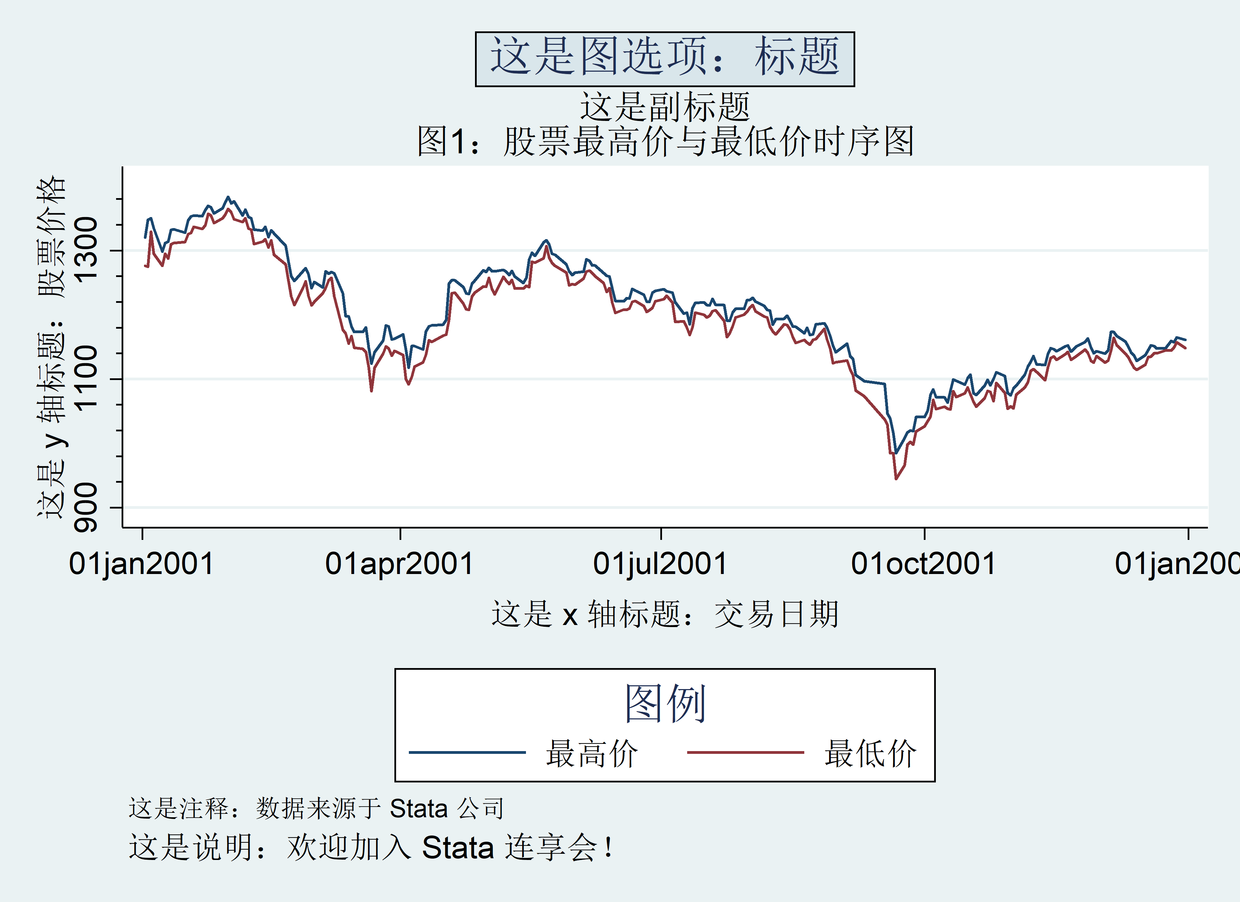#### 1.4 图形管理

``````/* 图形管理 */

*- 图形保存
sysuse sp500, clear
twoway line high low date, ///
saving(fig1.gph, replace) // 保存方式1
graph use fig1.gph // 重现图形

twoway line high low date, scheme(s1mono)
graph save fig2.gph, replace
// 保存方式2，scheme 是设定绘图模板

*- 图形合并
graph combine fig1.gph fig2.gph, col(1)
// 以单列形式合并

help graph combine

*- 图形导出
graph export "fig3.png", replace
// 导出格式有 png，tif，wmf 等
graph export "fig4.png", ///
width(3200) height(1800) replace
/* 调整输出图片的分辨率，

help graph export
``````

### 2. 绘图范例

#### 2.1 初识数据

``````******* 让我们开始吧
use "http://www.princeton.edu/~otorres/wdipol.dta", clear  // 导入数据
browse

describe
summarize
/* 了解数据结构
year:    年份
country: 国家名称
gdppc:   人均GDP
unempf:  女性失业率（%）
unempm:  男性失业率（%）
unemp:   失业率（%）
export:  出口额
import:  进口额
polity:  Polity IV数据库中的政体类型变量（polity）,
衡量政体的民主程度，
数值越大表示政体民主程度越高。
polity2: Polity IV数据库中的政体类型变量（polity2）,
衡量政体的民主程度，
数值越大表示政体民主程度越高。
id:      可通过命令group(country)得到，
将国家名称与数字相对应
*/
``````

#### 2.2 线图

• `lpattern(...)` 改变线条类型(如实线、虚线、点线等)。
• `lpattern(solid)` 表示将线条定义为实线。
• 可以借助命令 `palette linepalette` 查看线型及对应代号。
• `msymbol(...)` 改变标记符号(如实心圆圈、实心菱形、实心正方形)。
• `msymbol(o)` 表示将标记符号定义为实心小圆圈。
• 可以借助命令 `palette symbolpalette` 查看标记符号及对应代号。

``````******* 线图
line unemp unempf unempm year
if country=="United States"
// 利用 if 条件，只画美国失业率的时序图

summarize unemp unempf unempm
replace unemp=. if unemp==0
replace unempf=. if unempf==0
replace unempm=. if unempm==0
// 将变量为0的值变成缺失值
summarize unemp unempf unempm
line unemp unempf unempm year ///
if country=="United States"

twoway line unemp unempf unempm year ///
if country=="United States", ///
title("Unemployment rate in the US, 1980-2012") ///
legend(label(1 "Total") ///
label(2 "Females") ///
label(3 "Males")) ///
lpattern(solid dash dot) ///
ytitle("Percentage")
/*

*/

twoway connected unemp unempf unempm year
if country=="United States", ///
title("Unemployment rate in the US, 1980-2012") ///
legend(label(1 "Total") ///
label(2 "Females") ///
label(3 "Males")) ///
msymbol(circle diamond square) ///
ytitle("Percentage")
/*

*/

twoway connected unemp year
if country=="United States" | ///
country=="United Kingdom" | ///
country=="Australia" | ///
country=="Qatar", ///
by(country, title("Unemployment")) ///
msymbol(circle_hollow)

twoway connected unemp year
if country=="United States" | ///
country=="United Kingdom" | ///
country=="Australia" | ///
country=="Qatar", ///
by(country) ///
title("Unemployment") ///
msymbol(circle_hollow)
/*

title("")放在by()里面，画出的图共用一个标题；
title("")放在by()外面，每个图一个标题
*/

twoway (connected unemp year
if country=="United States", ///
msymbol(diamond_hollow)) ///
(connected unemp year
if country=="United Kingdom", ///
msymbol(triangle_hollow)) ///
(connected unemp year
if country=="Australia", ///
msymbol(square_hollow)) ///
(connected unemp year ///
if country=="Qatar", ///
title("Unemployment") ///
msymbol(circle_hollow) ///
legend(label(1 "USA") label(2 "UK") ///
label(3 "Australia") label(4 "Qatar")))
/*

*/

twoway connected gdppc year if gdppc>40000, ///
by(country) msymbol(diamond)
/* 保留人均GDP高于40000美元的子样本，再按国家分组绘制时序图*/

bysort year: egen gdppc_mean=mean(gdppc)
bysort year: egen gdppc_median=median(gdppc)
// 利用bysort命令，计算出人均GDP每年的平均数和中位数
twoway connected gdppc gdppc_mean year ///
if country=="United States" | ///
country=="United Kingdom" | ///
country=="Australia" | ///
country=="Qatar", ///
by(country, title("GDP pc (PPP, 2005=100)")) ///
legend(label(1 "GDP-PC") ///
label(2 "Mean GDP-PC")) ///
msymbol(circle_hollow)
/*

*/

help twoway line // 查看线图的帮助文件
help twoway connected // 查看点线图的帮助文件

palette symbolpalette // 图示标记符号及对应代号
palette linepalette // 图示线型及对应代号
palette color green // 图示颜色
help palett
``````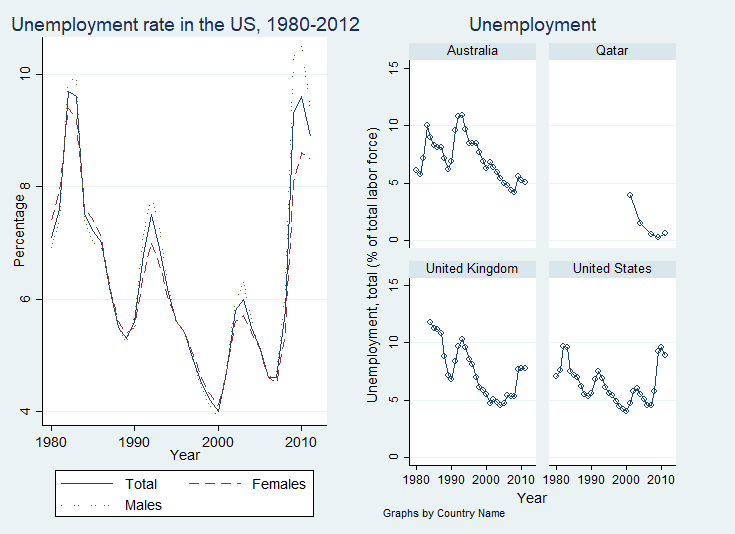#### 2.3 条形图

• `over(...)` 表示按什么变量进行分组。
• 有几个 `over`，就分几层。若有多个 `over`，出现顺序不一样，画出的图就不同。
• 比如，`over(var, sort(#) bargap(#) bar(#, color(red)))` 表示按变量 var 进行分组，根据第 # 个变量的柱体高度进行升序排列。
若要降序排列，命令则为 `over(var, sort(#) descending)`
• `bargap(#)` 设定组内条形之间的空隙大小；默认为 bargap(0)，表示组内条形之间紧密相连。
若设置参数为 `bargap(20)`，则表示组内条形之间所留的空隙为条形宽度的20%。
• `bar(#, color(red))` 单独设置第 # 个柱体的颜色。
• 可参考：教你如何一步一步绘制漂亮的分组条形图

``````*******  条形图
graph hbar (mean) gdppc
// 绘制横向条形图，平均值mean选项是默认值
graph hbar (mean) gdppc, ///
over(country, sort(1) descending)
graph hbar (mean) gdppc, ///
over(country, sort(1) ///
descending label(labsize(*0.5)))

graph hbar (mean) gdppc (median) gdppc ///
if gdppc>40000, ///
over(country, sort(1) descending ///
label(labsize(*1))) ///
legend(label(1 "GDPpc (mean)") ///
label(2 "GDPpc (median)"))
/*
over() 设定分组变量，这里表示按国家分组
sort(1) 选项表示根据第一个变量，
即 gdppc 的柱体高度进行升序排列
descending 表示降序排列
labsize(*0.5) 表示标签字体大小缩放 0.5 倍
*/

help graph bar // 查看条形图的帮助文件
``````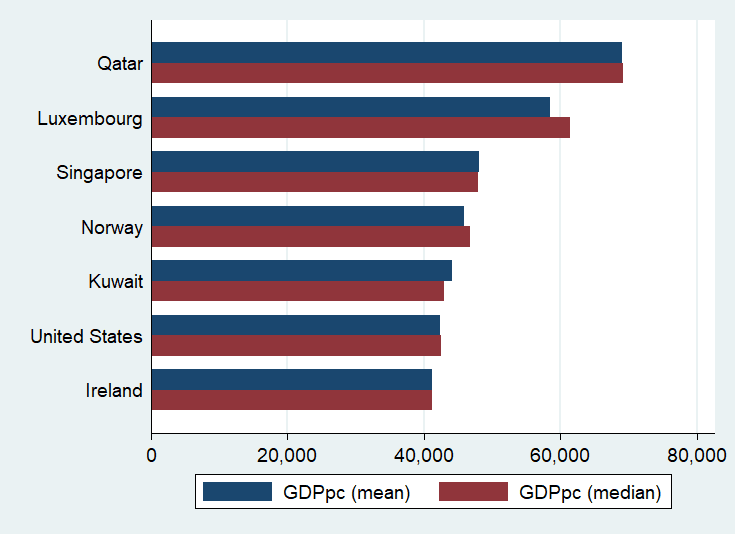#### 2.4 箱型图

• `marker(...)` 设定如何标记箱型图的异常值。
• 比如 `marker(#, msymbol(Oh) msize(small))` 以较小的空心圆 (Oh)，标识出第 # 个变量的特异值。

``````******* 箱形图
sort id year  // 排序，规范样本
recode polity2 (-10/-6=1 "Autocracy") ///
(-5/6=2 "Anocracy") ///
(7/10=3 "Democracy") ///
(else=.), ///
gen(regime) label(polity_rec)
/*

1 "威权政体" if -10<=polity2<=-6
2 "中间政体" if -5<=polity2<=6
3 "民主政体" if 7<=polity2<=10
*/

tab regime
// 对类别变量 regime 列表统计，结果包括频数，频率
tab regime, nolabel
// 列表统计，不显示类别变量的标签
tab country regime // 二维列表
tab country regime, row
// row 选项表示行末增加 Total 统计量

help tab

graph hbox gdppc // 绘制横向箱型图
graph hbox gdppc if gdppc<40000
graph box gdppc, over(regime) yline(4517.94) ///
marker(1, msymbol(Oh) msize(small))
/*
over(regime) 表示按regime（政体类型）分组
yline(4517.94) 添加附加线，即 y=4517.94 的直线
marker(1, msymbol(Oh) msize(small)) 以较小的空心圆，标识出第1个变量的特异值
*/

help graph box // 查看箱型图的帮助文件
``````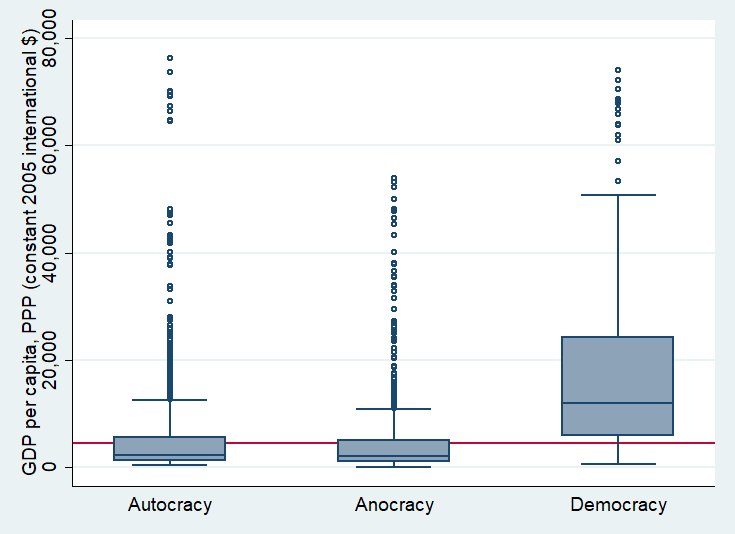#### 2.5 散点图

• `mlabel(varname)` 给散点添加文字标签。
• 比如 `mlabel(country)` 表示标上国家名称，注意 country 是已设定好的文字变量。
• `msymbol(...)` 改变标记符号(如实心圆圈、实心菱形、实心正方形)。
• 比如，`msymbol(o)` 表示将标记符号定义为实心小圆圈。
• 可以借助命令 `palette symbolpalette` 查看标记符号及对应代号。

``````******* 散点图
scatter import export // 进口额与出口额的散点图

twoway (scatter import export ///
if export>1000000, mlabel(country)) ///
(scatter import export), legend(off)

twoway (scatter import export) ///
(scatter import export ///
if export>1000000, mlabel(country)), legend(off)
/*
mlabel(country) 标上国家名称
legend(off) 不显示图例

*/

twoway (scatter import export, ///
ytitle("Imports") xtitle("Exports")) ///
(scatter import export ///
if export>1000000, mlabel(country) legend(off)) ///
(lfit import export, ///
note("Constant values, 2005, millions US\$"))
/*lfit 线性回归拟合图*/

capture bysort year: egen gdppc_mean=mean(gdppc)
// 利用bysort命令，计算出人均GDP每年的平均数;
// capture避免程序因错误而中断

twoway (scatter gdppc year, jitter(13)) ///
(connected gdppc_mean year, ///
msymbol(diamond)) , ///
xlabel(1980(1)2012, angle(90))
/*
jitter(#) 选项表示添加白噪声数据点，

xlabel(1980(1)2012) 设定横坐标刻度标签，
x轴的刻度从1980开始，到2012结束，每隔1添加一个刻度；
angle(90) 设定刻度标签的角度
*/

help twoway scatter // 查看散点图的帮助文件
``````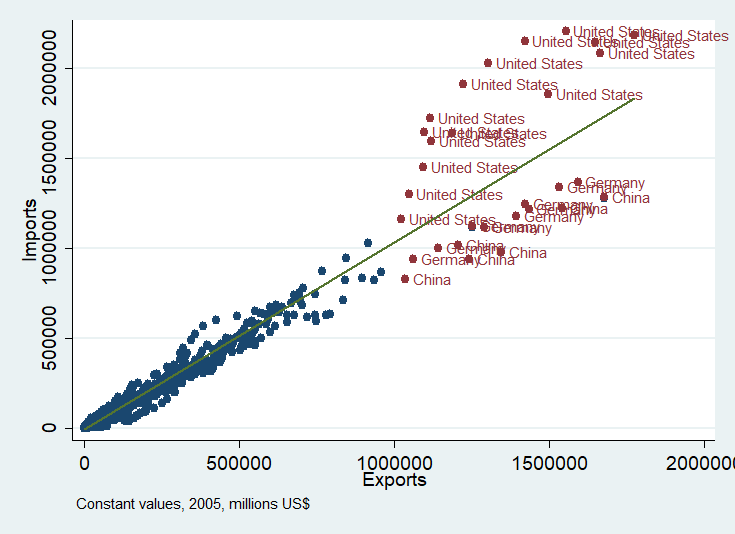#### 2.6 矩阵图

• `half` 表示只显示矩阵的一半，即左下角。
• `maxes(...)``maxis(...)`，两者等价，可用来控制 y 轴、x 轴刻度标签。
• 比如 `maxes(ylabel(none) xlabel(none))` 表示不显示 y 轴、x 轴刻度及标签。
• 比如 `maxes(ylabel(, nolabels))` 不显示 y 轴标签，但显示刻度。
• 比如 `maxes(ylabel(,grid) xlabel(,grid))` y 轴、x 轴均增加网格线。

``````******* 矩阵图
graph matrix gdppc unemp unempf unempm ///
maxis(ylabel(none) xlabel(none))

graph matrix gdppc unemp unempf unempm ///
half maxis(ylabel(none) xlabel(none))
/*
graph matrix 命令用矩阵的形式

half 表示只显示矩阵的一半，即左下角。
maxis(ylabel(none) xlabel(none))

*/

help graph matrix // 查看矩阵图的帮助文件
``````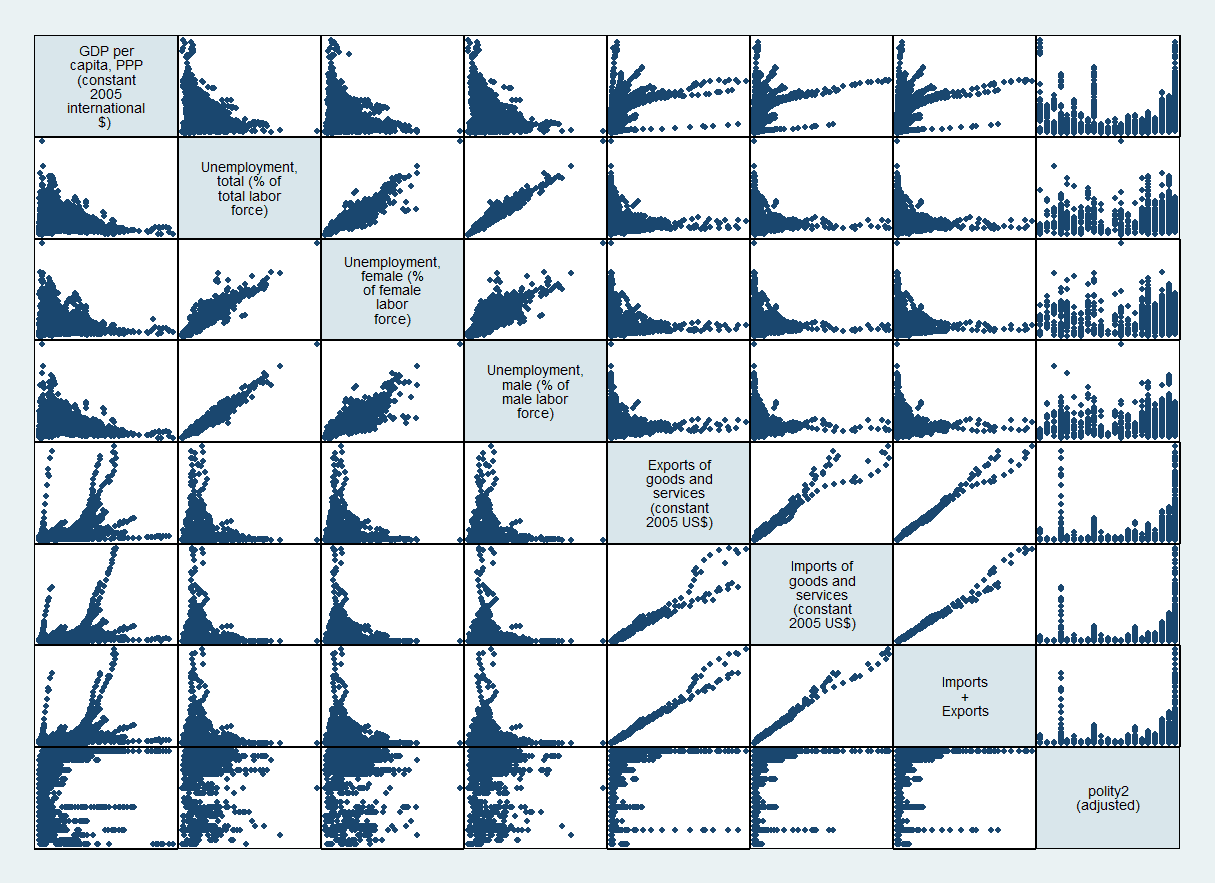#### 2.7 直方图

• `bin(#)` 指定分为 # 个组别。
• 比如 `bin(10)` 将连续变量分为10个组别。
• `barwidth(#)` 控制直方图柱子的宽度。
• `fcolor(...)` 设定柱子的填充颜色。
• 比如 `fcolor(none)` 表示无填充颜色。
• 可通过外部命令 `palette_all` 查看颜色代号。运行 `ssc install palette_all` 即可下载该外部命令。
• 可通过外部命令 `full_palette` 查看 66 种颜色及其 RGB 代码。运行 `ssc install full_palette` 即可下载该外部命令。
• `lcolor(...)` 设定柱子的轮廓颜色。
• 比如 `lcolor(black)` 设定柱子的轮廓颜色为黑色。
• `lwidth(...)` 设定外边缘线的宽度。
• 比如 `lwidth(medium)` 设定外边缘线的宽度为适中。
• 可通过命令 `graph query linewidthstyle` 列示线宽代号。
• `lpattern(...)` 设定外边缘线的类型。
• 比如 `lpattern(dash)` 设定外边缘线为虚线。
• 可通过命令 `palette linepalette` 图示线型代号。

``````******* 直方图
hist gdppc // 频率分布
hist gdppc, frequency // 频数分布
hist gdppc, kdensity
// 将直方图和核密度曲线绘制在一起
hist gdppc, kdensity normal
// 同时显示频率直方图、核密度曲线和正态分布图
hist gdppc, kdensity normal bin(20)
// bin(#)指定分为几个组别
hist gdppc if country=="United States" | ///
country=="United Kingdom", ///
bin(10) by(country)
// 利用by(varname)设定分组，同时画多个图。

twoway hist gdppc ///
if country=="United States", bin(10) || ///
hist gdppc ///
if country=="United Kingdom", bin(10) ///
fcolor(none) lcolor(black) ///
lwidth(medium) lpattern(dash) ///
legend(label(1 "USA") label(2 "UK"))
/*
fcolor(none) 设定柱子的填充颜色，none表示无填充颜色
lcolor(black) 设定柱子的轮廓颜色
legend()设置图例
lwidth(medium) 设定外边缘线的宽度
lpattern(dash) 设定外边缘线的类型
*/

help linewidthstyle
help linepatternstyle
help hist // 查看直方图的帮助文件
``````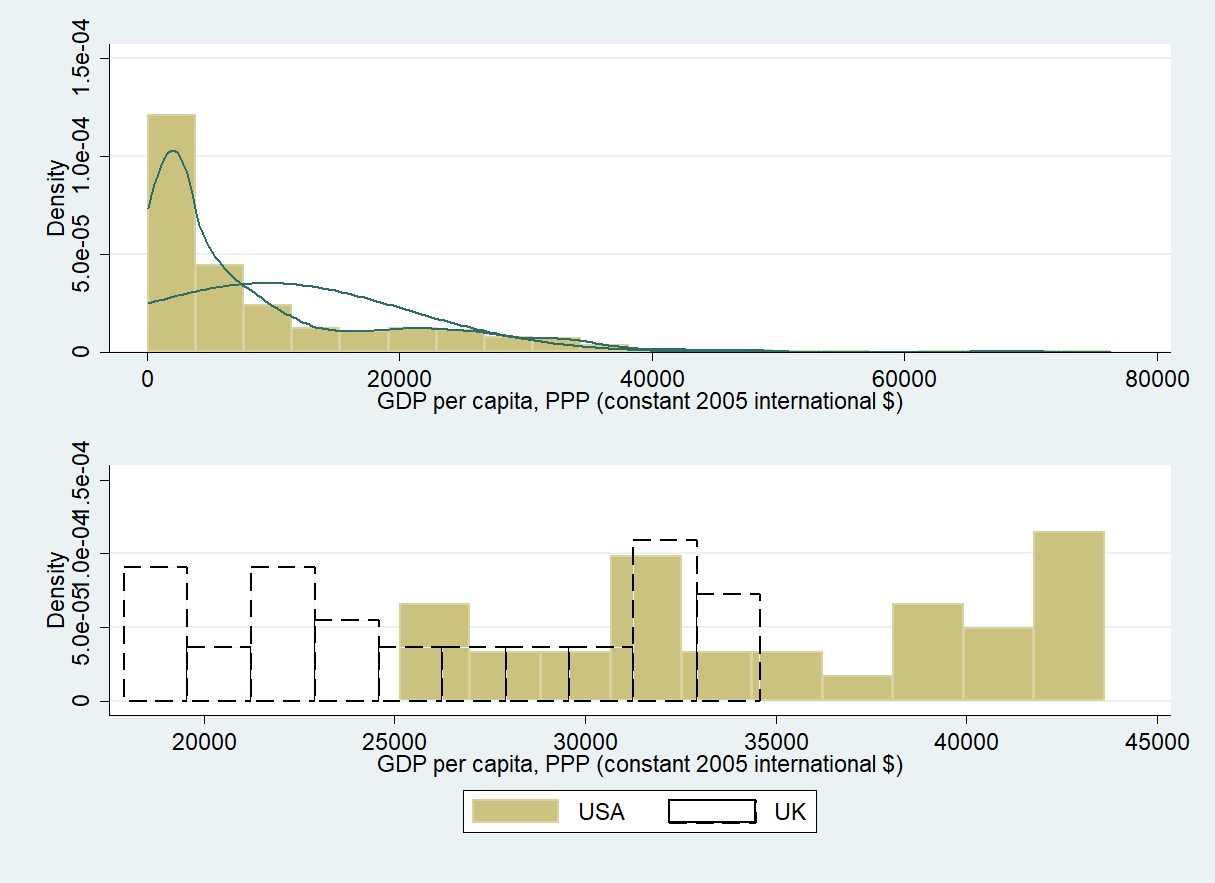#### 2.8 面板数据时间趋势图

``````******* 面板数据时间趋势图
*xtset country year
// 会报错，'country' 为字符串变量*/
encode country, gen(country1)
xtset country1 year // 声明数据是面板数据

xtline gdppc
xtline gdppc if gdppc>39000, overlay
// overlay 将所有国家放在同一图中

help xtline // 查看面板数据时间趋势图的帮助文件
``````

#### 2.9 点图

``````******* 点图
graph dot (mean) gdppc if gdppc>40000, ///
over(country, sort(1) descending)

graph dot (mean) gdppc (median) gdppc ///
if gdppc>40000, ///
over(country, sort(1) descending label(labsize(*1))) ///
legend(label(1 "GDPpc (mean)") label(2 "GDPpc (median)"))
// * 事实上是柱状图的另一种表示方法, 比较省墨

help graph dot // 查看点图的帮助文件
``````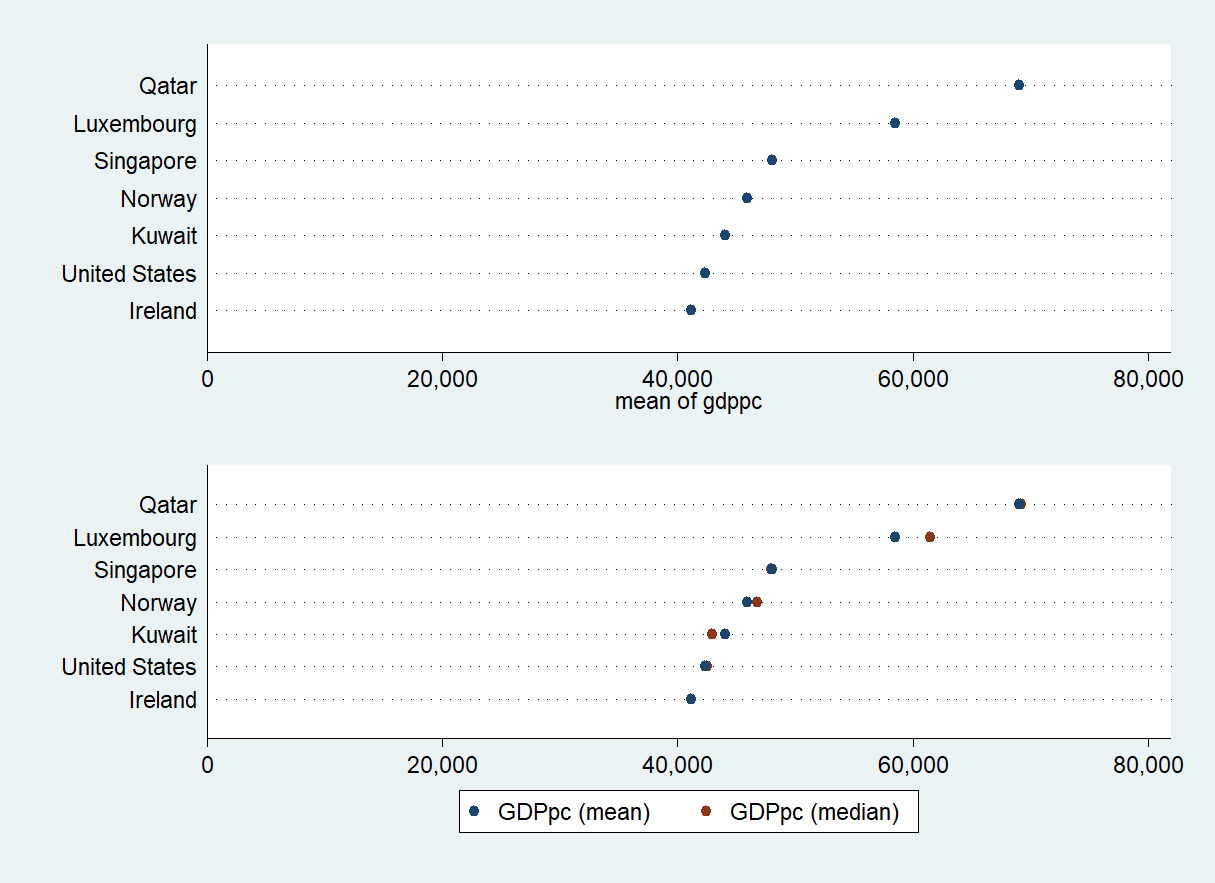#### 2.10 饼图

• `plabel(...)` 控制饼块的标签。
• 比如 `plabel(_all percent,format("%5.2f"))` 为所有饼块按 %5.2f 格式显示百分比。
• 比如 `plabel(_all sum)` 为所有饼块显示总数。
• 比如 `plabel(_all name)` 为所有饼块显示类别名。
• 比如 `plabel(1 percent,gap(20))` 为第一饼显示百分比,其中 gap(#) 控制标签距离圆心的相对距离。
• `pie(...)` 设置饼块的颜色，以及是否突出/分离。
• 比如 `pie(#,explode)` 突出/分离第 # 饼块。
• 比如 `pie(#, color(red))` 令第 # 饼块的颜色为红色。

``````******* 饼图
graph pie export if     ///
(country=="Brazil" | ///
country=="Russia" | ///
country=="India"  | ///
country=="China") & year == 2010, ///
over(country) noclockwise
// noclockwise 逆时针排序

graph pie export if     ///
(country=="Brazil" | ///
country=="Russia" | ///
country=="India"  | ///
country=="China") & year == 2010, ///
over(country) sort descending

graph pie export if     ///
(country=="Brazil" | ///
country=="Russia" | ///
country=="India"  | ///
country=="China") & year == 2010,    ///
over(country) sort descending        ///
plabel(_all percent,format("%5.2f")) ///
pie(1,explode)

/*
sort descending 降序排列; sort 升序排列
plabel(_all percent,format("%7.2f"))

pie(1,explode)  突出/分离第1块饼块
*/

help graph pie // 查看饼图的帮助文件
``````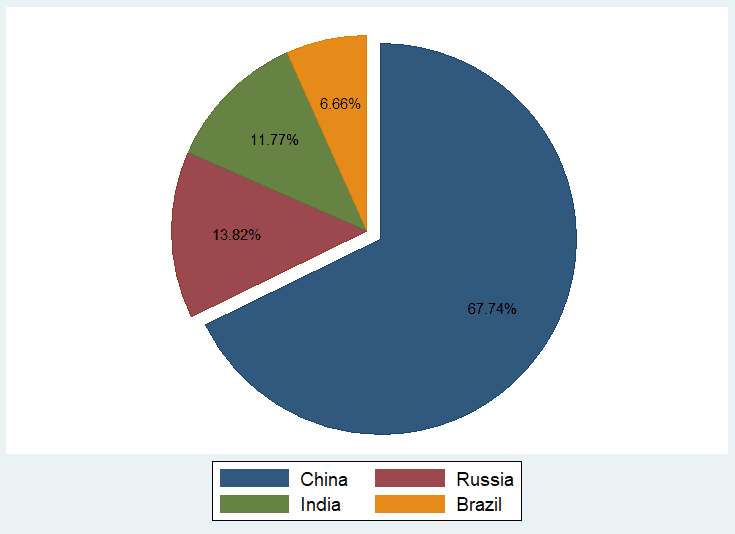### 参考资料

1. Intro to data visualization
2. 陈传波 《Stata十八讲》

#### 关于我们

• 「Stata 连享会」 由中山大学连玉君老师团队创办，定期分享实证分析经验， 公众号：StataChina
• 公众号推文同步发布于 CSDN简书知乎Stata专栏。可在百度中搜索关键词 「Stata连享会」查看往期推文。
• 点击推文底部【阅读原文】可以查看推文中的链接并下载相关资料。
• 欢迎赐稿： 欢迎赐稿。录用稿件达 三篇 以上，即可 免费 获得一期 Stata 现场培训资格。
• E-mail： [email protected]
• 往期推文：计量专题 || 精品课程 || 简书推文 || 公众号合集